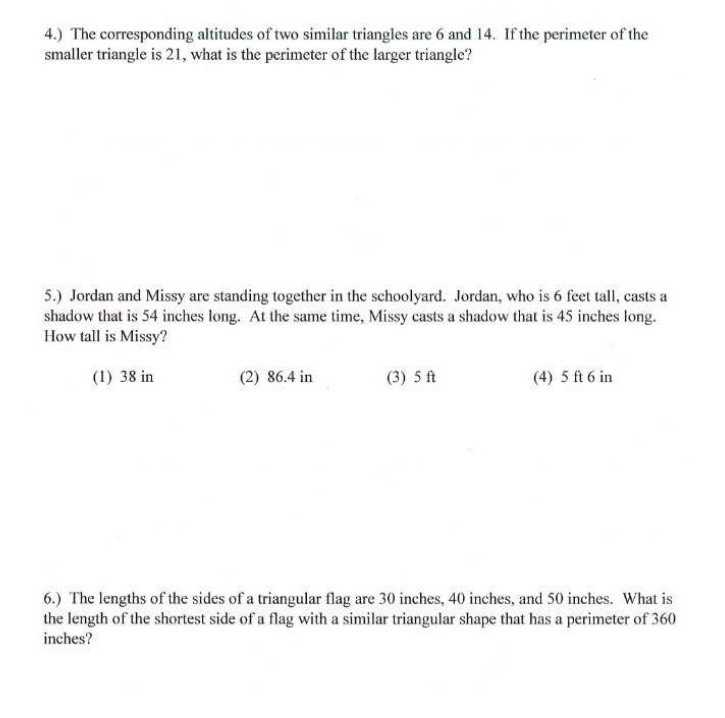### ¿Todavía tienes preguntas de matemáticas?

Pregunte a nuestros tutores expertos
Algebra
Pregunta4.) The corresponding altitudes of two similar triangles are $$6$$ and $$14$$ . If the perimeter of the smaller triangle is $$21$$ , what is the perimeter of the larger triangle?

5.) Jordan and Missy are standing together in the schoolyard. Jordan, who is $$6$$ feet tall, casts a shadow that is $$54$$ inches long. At the same time, Missy casts a shadow that is $$45$$ inches long. How tall is Missy? (1) $$38$$ in (2) $$86.4$$ in

6.) The length of the shortest side of a flag with a similar triangular shape that has a perimeter of $$360$$ inches?

$$49\\5\\90$$